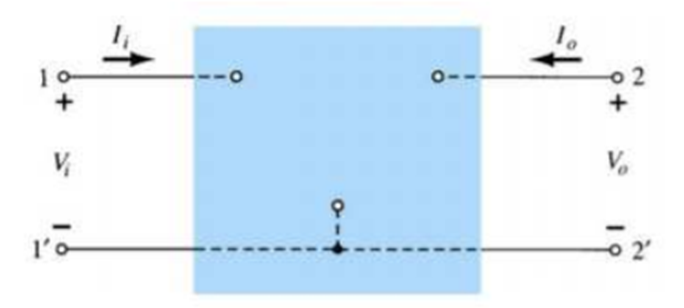Goseeko blog# What is the CB Hybrid Model?

We use h-parameter model to analyze the Small signal response. Before moving on to the CB (common base) hybrid model we first understand about the hybrid model. We then first derive the general expression of various transistor parameters in terms of hybrid parameters. Later in this article we will derive expressions of the CB (common base) hybrid model.

The hybrid two port model is shown below.Vi = h11Ii + h12Vo

Io = h21Ii + h22Vo

h11 = hi = Vi/Io for Vo =0  [Input resistance]

Reverse voltage gain

h12 = hr = Vi/Vo for Ii =0

Then Forward current gain

h21 = hf = Io/Ii for Vo =0

Hence,

h22 = ho = Io/Vo for Ii =0   [output admittance]

The hybrid equivalent model is shown below

The transistor model has three terminals with two ports.

Input resistance = hi

Output conductance= ho

The reverse transfer voltage

hr= Vi/Vo

hf= Io/Ii = forward transfer current ratio

The simplified model is shown below

## Simplified Hybrid Model parameters

Finding current gain Ai

Applying KCL at the output of above circuit

Io =I + hf Ib = Vo ho + hf Ib

Vo = -Io RL

Then

Io = -Io RL ho + hf Ib

Io (1 + RL ho) = hf Ib

Ai = Io/ Ib = hf/(1 + RL ho)

Finding Voltage Gain AV

Applying KVL at input of the above h-model

Vi = hi Ib + hr Vo

But Ib = (1 + ho RL)/hf

Io = – Vo/RL

Substituting in above equation and solving for Vo/Vi­­ we get

Av = – hf RL/hi + (hi ho– hf hr) RL

Finding Input Impedance Zi

Vi = hi Ib + hr Vo

Then

Io = – Vo/RL

Ai = Io/ Ib

Since

Vi = hi Ib – hrRLAiIb

Hence the input impedance is

Zi = Vi/Ib = hi – hrRLAi

Finding Output impedance Zo

It is ratio of output voltage to output current with Vs =0. Then the value of output current becomes

Io = Voho + hfIb

Ii = -hrVo/Rs + hi

Zo = Vo/Io = 1/[ho – (hf h r /hi+ Rs)]

## CB hybrid Model Parameters

The common base configuration hybrid model is also shown below. It is the hybrid equivalent of a CB transistor. As we also know input in common base transistors is applied between base emitter terminals. Then the output is collected from the collector emitter junction. Then value of input voltage Vbe and output current IC are as

Vbe =hib ib +hrb VC

ie = hfbib + hobVC

The hybrid expression can also be obtained from the general hybrid formula discussed above by adding a second subscript letter b which commonly stands for base with the h-parameters.

### Current Gain

As derived earlier the value of current gain for CB configuration will be

Ai = -(hfb / (1+ hob rL)

But rL = Rc|| RL

Since hfb is a positive number therefore Ai of a CE amplifier is negative.

### Input Resistance

Then value of resistance at the input terminal in the figure is

Ri = hib+ hrb Ai rL= hib – ((hrbhfb )/ (hob + (1/rL)))

The input resistance of the amplifier stage also depends upon the biasing arrangement. Then a fixed biased the stage input resistance is,

Ris= R

### Voltage Gain

Av = Ai  r1 /Ri

We also know that common base amplifiers have positive current gain. Hence, the voltage gain is also positive. Therefore, the output and input are in phase. Then h-parameter is

Av = hfb  rL /(hib + Δh rL)

Δh = hib  hob – hrb  hfb

### Output Resistance

Ro = Rs  + hib  /(Rs hob + Δh)

Δh = hib  hob – hrb  hfb

Overall Voltage Gain

Avs = Av  Ris /(Rs + Ris)

Overall Current Gain

Ais = Avi Rs /(Rs + Ris)